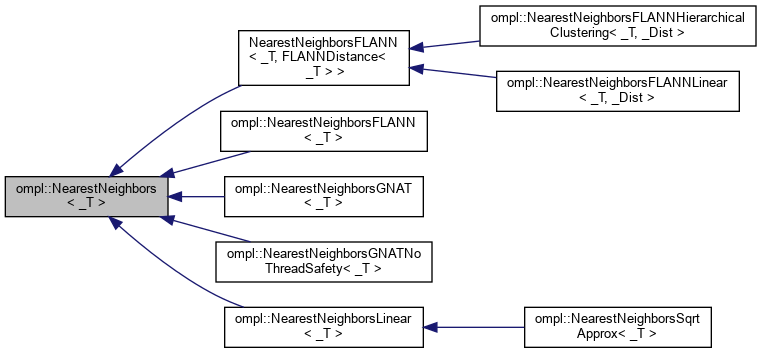ompl::NearestNeighbors< _T > Class Template Referenceabstract

Abstract representation of a container that can perform nearest neighbors queries. More...

#include <ompl/datastructures/NearestNeighbors.h>

Inheritance diagram for ompl::NearestNeighbors< _T >:[legend]

Public Types

using DistanceFunction = std::function< double(const _T &, const _T &)>
The definition of a distance function.

Public Member Functions

virtual void setDistanceFunction (const DistanceFunction &distFun)
Set the distance function to use.

const DistanceFunctiongetDistanceFunction () const
Get the distance function used.

virtual bool reportsSortedResults () const =0
Return true if the solutions reported by this data structure are sorted, when calling nearestK / nearestR.

virtual void clear ()=0
Clear the datastructure.

virtual void add (const _T &data)=0
Add an element to the datastructure.

virtual void add (const std::vector< _T > &data)

virtual bool remove (const _T &data)=0
Remove an element from the datastructure.

virtual _T nearest (const _T &data) const =0
Get the nearest neighbor of a point.

virtual void nearestK (const _T &data, std::size_t k, std::vector< _T > &nbh) const =0
Get the k-nearest neighbors of a point. More...

virtual void nearestR (const _T &data, double radius, std::vector< _T > &nbh) const =0
Get the nearest neighbors of a point, within a specified radius. More...

virtual std::size_t size () const =0
Get the number of elements in the datastructure.

virtual void list (std::vector< _T > &data) const =0
Get all the elements in the datastructure.

Protected Attributes

DistanceFunction distFun_
The used distance function.

Detailed Description

template<typename _T> class ompl::NearestNeighbors< _T >

Abstract representation of a container that can perform nearest neighbors queries.

Definition at line 47 of file NearestNeighbors.h.

◆ nearestK()

template<typename _T >
 virtual void ompl::NearestNeighbors< _T >::nearestK ( const _T & data, std::size_t k, std::vector< _T > & nbh ) const
pure virtual

Get the k-nearest neighbors of a point.

All the nearest neighbor structures currently return the neighbors in sorted order, but this is not required.

◆ nearestR()

template<typename _T >
 virtual void ompl::NearestNeighbors< _T >::nearestR ( const _T & data, double radius, std::vector< _T > & nbh ) const
pure virtual

Get the nearest neighbors of a point, within a specified radius.

All the nearest neighbor structures currently return the neighbors in sorted order, but this is not required.

The documentation for this class was generated from the following file: